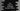# C++ program to find if n factorial is divisible by sum of first n numbers or not## C++ program to find if n factorial is divisible by sum of first n numbers or not:

In this post, we will learn how to find the n factorial is divisible by sum of first n numbers or not. This program will take the value of n as input from the user and print one message that it is divisible by the sum of first n or not.

### Algorithm:

Our program will take the value of n and it will use two different functions to:

• Calculate the factorial.

• Factorial of a number is equal to the product of all numbers from 1 to n. So, we will write one function that will find the products from 1 to n.
• Calculate the sum from 1 to n.
• Check if both of these values are equal or not.

### C++ program:

Let’s write down the program:

``````#include <iostream>
using namespace std;

int findFactorial(int n)
{
int factorial = 1;

for (int i = 1; i <= n; i++)
{
factorial *= i;
}

return factorial;
}

int findSum(int n)
{
return n * (n + 1) / 2;
}

int main()
{
int n;

cout << "Enter the value of n: " << endl;
cin >> n;

int factorial = findFactorial(n);
int sum = findSum(n);

if (sum == factorial)
{
cout << "Both sum and factorial values are equal" << endl;
}
else
{
cout << "Sum and factorial values are not equal" << endl;
}
}``````

Here,

• findFactorial method is used to find the factorial for a number. It takes one int value as the parameter and returns the factorial for that number.

• This method creates one integer variable factorial initialized as 1.
• It runs one for loop from i = 1 to i = n.
• On each iteration, it multiplies the value of i with factorial.
• Once the loop ends, factorial variable holds the factorial of n.
• findSum method is used to find the sum of 1 to n. It takes the value of n and returns the sum.
• The program is taking the value of n as an input from the user and storing that value in the variable n.
• It calculates the factorial and sum, stores these values in factorial and sum integer variables.
• The final if-else block check if both sum and factorial are equal or not. Based on that, it prints one message.

### Sample output:

If you run this program, it will print output as like below:

``````Enter the value of n:
3
Both sum and factorial values are equal

Enter the value of n:
10
Sum and factorial values are not equal``````